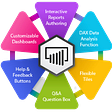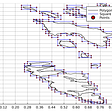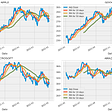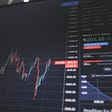# Highlights

• Difficulty：★★☆☆☆

# Preface

when the United States 10Y Government Bond -United States 2Y Government Bond <0 ,we call it yield curve inversion 。

Usually longer-term bonds will have higher interest rates, but when the market have doubts about the economy ,yield cruve inversion happen! So in this article , we try to plot the Macroeconomic Index a year before it happened, and two years after it happened

# The Editing Environment and Modules Required

Window10 Spyder(anaconda31)

# Database

macroeconomic data :macroeconomic data database code (GLOBAL/ANMAR)

`#######################3from datetime import datetime, timedelta import pandas as pdimport numpy as npimport matplotlib.pyplot as plt #########################3import tejapiimport plotly.graph_objects as goimport plotly.io as pio##################pio.renderers.default = 'browser'       ##PLOT IN WEB`

# Data Processing

our bonds data is from Tej pro

`df=pd.read_excel("C:\\Users/user/Desktop/Tej/美國公債殖利率.xlsx")`

There is Nan in our data , so we should drop it ,After we reset index

`df.dropna(inplace=True)df.reset_index(drop=True,inplace=True)`

# step 1 set the indicator

`df["dif2Y"]=df.US10Y-df.US2Ydf["invertkeepday"]=df["dif2Y"].apply(lambda x : 1 if x < 0 else 0 )df["cross"]=np.nandf["notcross"]=np.nan`

calculate the 10y- 2y

find the inverse day and visualize it

`startday=[]endday  =[]keep = 0 df=df.copy()for i in range(len(df)):    if df["invertkeepday"][i]  >0 :        if keep == 0:                    df["cross"][i]=df["US10Y"][i]            startday.append(df["日期"][i])            keep = 1    elif  keep == 1 :        df["notcross"][i]=df["US10Y"][i]if  df["invertkeepday"][i] < 1 :            endday.append(df["日期"][i])            keep = 0`

and we want to see how long did it happen

`for start , end  in zip(startday,endday):print(start , end, end-start)`

We found that some times were really close ,so we make them in a group

`inverted=pd.DataFrame(endday,startday)inverted.reset_index(inplace=True)inverted["year"]=inverted["index"].dt.yeardatetime=inverted.groupby('year')datetime=list(datetime)getstart=[]getend  =[]for i  in datetime :    i.reset_index(drop=True,inplace=True)    start = i["index"]    end = i[ 0][len(i)-1]    getstart.append(start)    getend.append(end)for start , end  in zip(getstart,getend):    print(start , end, end-start)`

Now we can see the approximately situation

Nearly three years we found that there have about 6 times yeild curve inverse

`df.set_index("日期",inplace=True)plt.figure(figsize=(12.2,4.5))plt.scatter(df.index,df['cross'],color='red', label='cross!',marker='x',alpha=1)plt.scatter(df.index,df['notcross'],color='green', label='Notcross',marker='o',alpha=1)plt.plot(df["US2Y"], label='2Y', alpha=0.35)plt.plot(df["US10Y"], label='10Y', alpha=0.35)plt.plot(df["dif2Y"], label='dif', alpha=0.35)plt.title('Close Price Buy & Sell Signals')plt.xticks(rotation=45)plt.xlabel('Date')  plt.ylabel('Price')plt.legend()plt.grid()`

# Step 2 visualize the stocks

we have the time so we can input the time into Tejapi to get the macroecnomic data

# Conclusion

From the perspective of various economic data, it may represent that the prosperity has passed its peak, so there are doubts about the future prosperity, selling short-term bonds and preferring longer-term bonds, which leads to the inversion of yield curves

Although every time an inversion occurs, it will be accompanied by an recession, but in these five expreience ,there was two times that the U.S. economy has not experienced a recession within two years

In 2019, the recesion happen because of the COVID-19 , yield curve can’t forecast it !

1, In the past 5 inversion ,stocks go up 4 time

2, When three recessions occurred, they were all in a rate-cut cycle, and when two recessions did not occur, the U.S. was in a rate-raising cycle

At present, although the peak of the US economic data has passed, it is still maintain at a very high level. Because of the strong economic performance, the US Federal Reserve has entered a cycle of raising interest rates. Although each inversion will be followed by a recession, this indicator It is a very leading indicator. The past five inversions between the 2 Y and the 10Y have taken an average of 18 months before a recession occurs. Perhaps the inversion is trying to tell us

Hey ! it’s time to be aware of the risks

You could give us encouragement by …
We will share financial database applications every week.
If you think today’s article is good, you can click on the
applause icononce.
If you think it is awesome, you can hold the
applause icon until 50 times.

# Source Code

--

--

--

## Interview with Kaggle GrandMaster: Dr Bojan Tunguz## Microsoft Power BI Features — Reasons Why Power BI is a Leader in its Field!## Using OntoRefine to Transform Tabular Data into Linked Data## iOS14: A proposed solution for the reduced efficacy of deterministic attribution## Computational cost-effective manipulation of geo boundaries## Weekly Report (21st Feb to 25th Feb)## AirBnB in Seattle Data Analytics## Data-Driven Legal Help## 台灣經濟新報(TEJ)

TEJ為台灣本土第一大財金資訊公司，成立於 1990 年，專門提供金融市場基本分析所需資訊，以及信用風險、法遵科技、資產評價、量化分析及ESG等解決方案及顧問服務。鑒於財務金融領域日趨多元與複雜，TEJ 結合實務與學術界的精英人才，致力於開發機器學習ML、人工智慧AI及自然語言處理NLP等新技術，持續提供創新服務

## Data Mining Team Proof of Value, April 2022## Stock Data Analysis and Portfolio Management## 【Data Analysis(13)】SVM Model